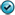## Exercises - Implicit Differentiation

1. Find $y'$ where

1. $\displaystyle{e^{yx} + \cos^2 x = y}$

2. $\displaystyle{xy^2 + y^3 - y = x^3 \tan y}$

3. $\displaystyle{\textrm{Arctan } (xy^2) - \frac{1}{y} = x^3}$

2. Find $\displaystyle{\frac{dy}{dx}}$

1. $\displaystyle{\cos(xy) = y^2 + 2x}$

2. $\displaystyle{x\sin y = y\cos x}$

3. $2y = x^2 + \sin(xy)$

4. $x^2+x^2y-3xy^2=2y^3+3$

3. Find $\displaystyle{\frac{dy}{dx}}$ and $\displaystyle{\frac{d^2y}{dx^2}}$ in simplest form

1. $x^2-4xy+2y^2=7$2. $xy = \ln(xy)$

4. Find $\displaystyle{\frac{d^2y}{dx^2}}$

1. $4y^2 = 3xy + x^2$

2. $x^2y^2 = 4$

3. $\sin(x^2 y) = -\cos(x^2y)$

5. Find the equations of the tangent lines to $(x+y)^2 = xy + 7$ at $x=3$

6. Show that $xy=1$ and $x^2 - y^2 = 1$ intersect at right angles

7. Find $\displaystyle{\frac{dy}{dx}}$ and evaluate the same at $(-1,2)$ if $x^3+y^3 = 2xy + 11$

8. Find the equation of the normal line to $\sqrt{x} - \sqrt{y} = y-3$ at $(9,4)$

9. Find $y'$ where $3x + y^2 = \sin xy + y$

10. Find $y''$ where $xy + y^2 = 1 + 2x$

11. Find the second derivative for $2x^2 - 3xy - y^2 = 1$ in simplest form.

12. Show that if $x^2-2xy-y^2=4$, then $\displaystyle{\frac{d^3y}{dx^3} = \frac{48x}{(x+y)^5}}$

13. Find the equation of the tangent line to $x^4y^2-4xy^5=x^3y^3$ at $(2,1)$.

14. Find the equation of the normal line to $x^2+xy-y^2 = 1$ at $(2,3)$.